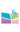﻿2020.01.03，楼森岳教授，宁波大学，A new 2+1 KdV equation from KP hierachy-中国矿业大学（北京）理学院网站2020.01.03，楼森岳教授，宁波大学，A new 2+1 KdV equation from KP hierachy

Starting from the master symmetry approach, one can find a nonlocal KP hierachy. The third and the fourth members of the hierachy are all the (2+1)-dimentional extensions of the KdV equation. Similarly, The nth and the (n+1)th members are the (2+1)-dimensional extensions of the nth order KdV equation equation. The multiple soliton solutions of the new (2+1)-dimensional KdV equations are explicitly reported.

间： 202013 下午4:15---5:00

点： 逸夫楼1537